# ELECTRICITY AND MAGNETISM 1 ELECTRICITY AND MAGNETISM SPS

• Slides: 23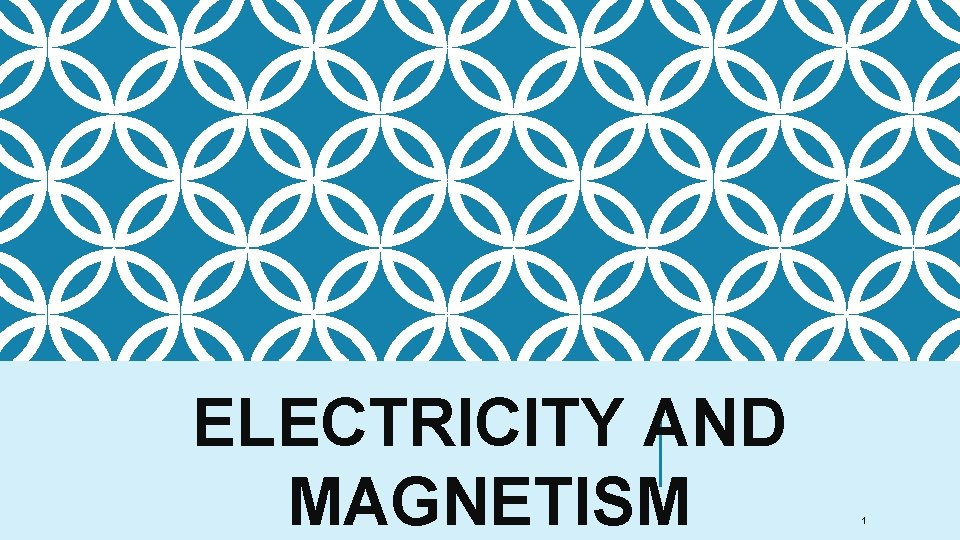ELECTRICITY AND MAGNETISM 1ELECTRICITY AND MAGNETISM SPS 10. Obtain, evaluate, and communicate information to explain the properties of and relationships between electricity and magnetism. a. Use mathematical and computational thinking to support a claim regarding relationships among voltage, current, and resistance. b. Develop and use models to illustrate and explain the conventional flow (direct and alternating) of current and the flow of electrons in simple series and parallel circuits. (Clarification statement: Advantages and disadvantages of series and parallel circuits should be addressed. ) c. Plan and carry out investigations to determine the relationship between magnetism and the movement of electrical charge. (Clarification statement: Investigations could include electromagnets, simple motors, and generators. )STATIC ELECTRICITY Static - some of the outer electrons are held very loosely. They can move from one atom to another. An atom that loses electrons has more protons than electrons. It is positively charged- cation An atom that gains electrons has more electrons than protons. It has a negative charge- anion. A charged atom is called an "ion. " 3STATIC ELECTRICITY Static electricity is the imbalance of positive and negative charges. 4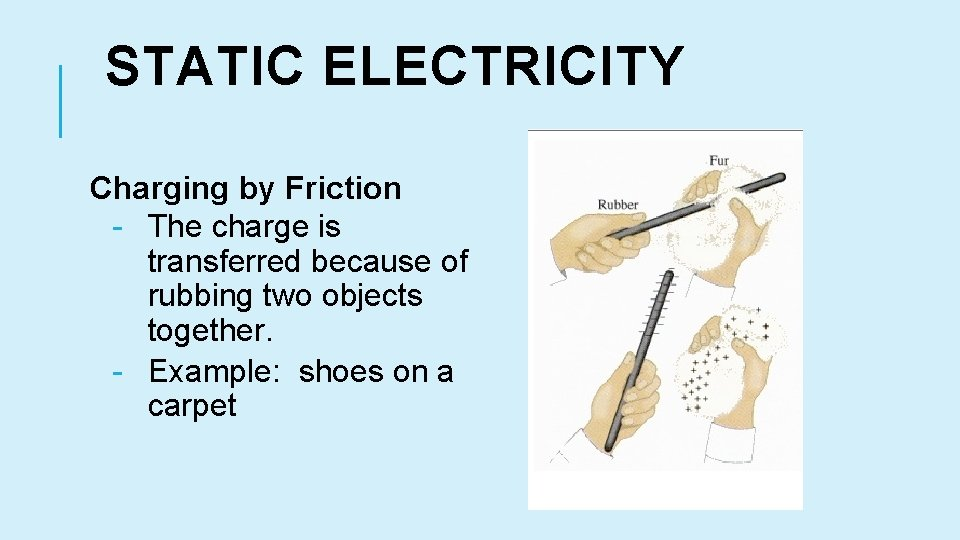STATIC ELECTRICITY Charging by Friction - The charge is transferred because of rubbing two objects together. - Example: shoes on a carpet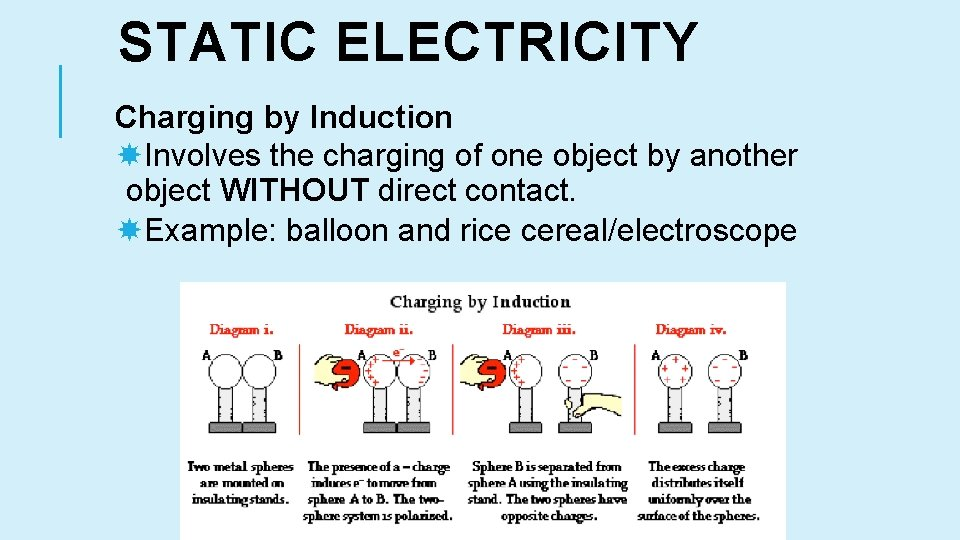STATIC ELECTRICITY Charging by Induction Involves the charging of one object by another object WITHOUT direct contact. Example: balloon and rice cereal/electroscopeSTATIC ELECTRICITY Charging by Conduction Involves the DIRECT CONTACT of a charged object to a neutral object. Example: Van de Graaf generatorELECTRICITY To make somethin" turn on, we need: an appropriate source of electricity (battery/outlet), metal wires insulated with plastic, a switch and the “thing” (refrigerator, light, computer, radio controlled car, sewing machine. . . ). We connect them in a distinct sequence for the thing to work. 8SOURCE OF ENERGY In the case of DC (Direct Current – (battery) current flows in one direction only), it has a limited life than is unusable so we throw it away. -In the case of AC (Alternating Current – (wall plug) current flows back and forth (changes direction)) the power company provides the electricity, it is far closer to limitless as an energy source. 9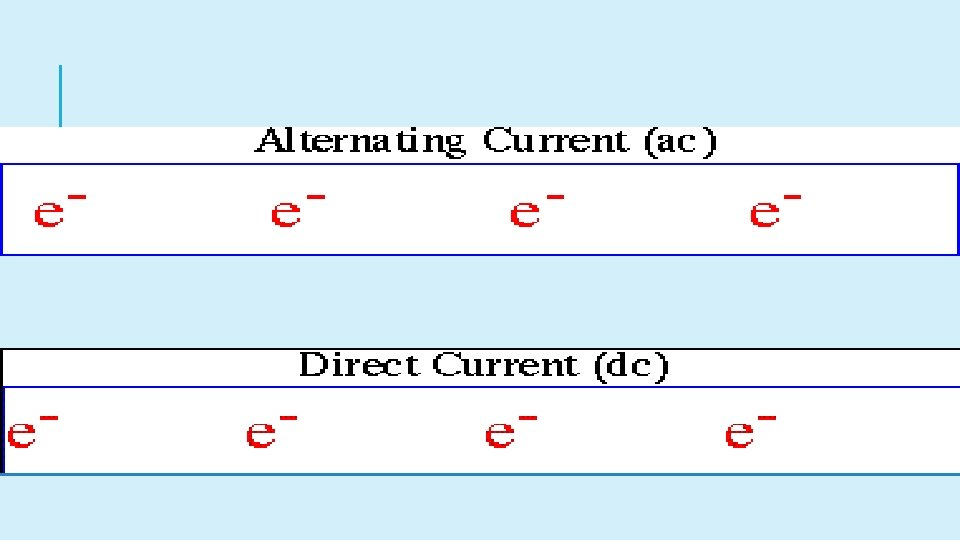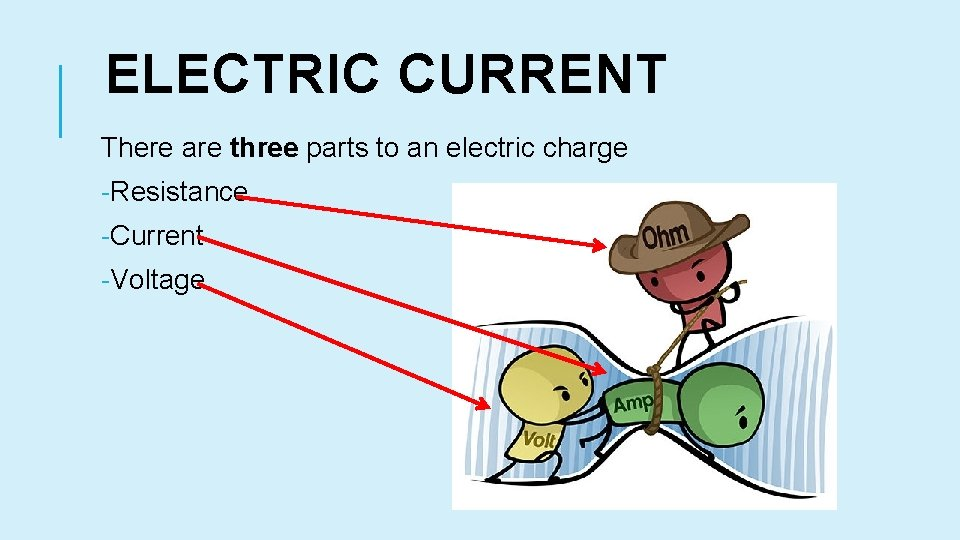ELECTRIC CURRENT There are three parts to an electric charge -Resistance -Current -Voltage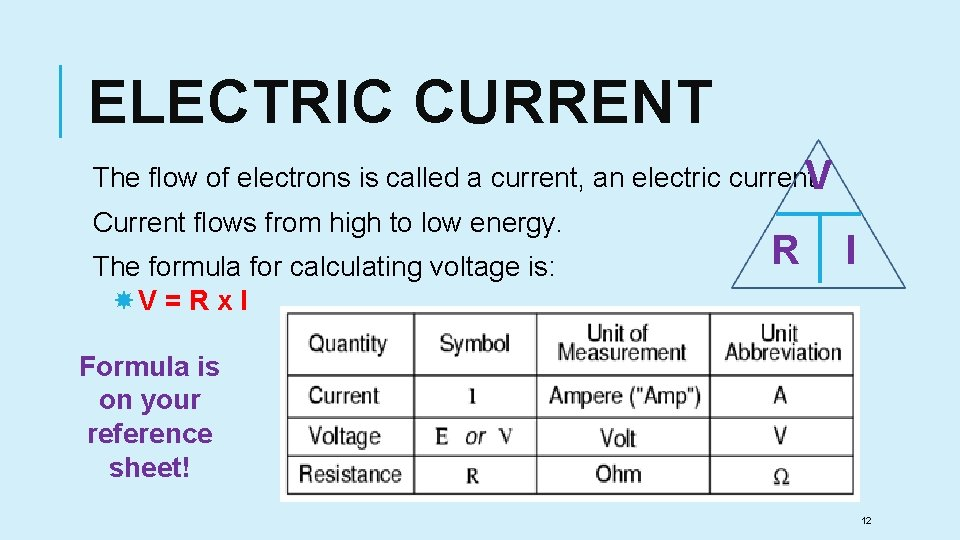ELECTRIC CURRENT The flow of electrons is called a current, an electric current. V Current flows from high to low energy. The formula for calculating voltage is: V = R x I R I Formula is on your reference sheet! 12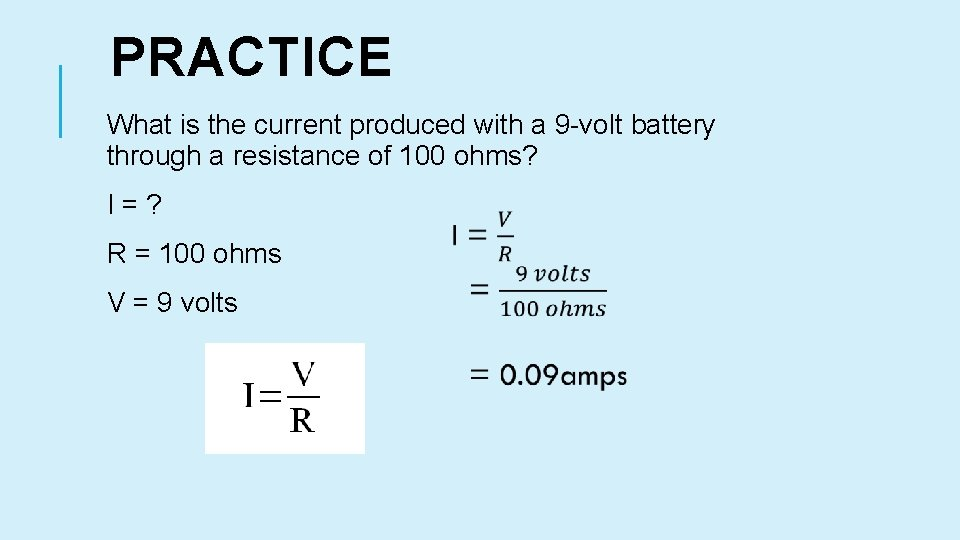PRACTICE What is the current produced with a 9 -volt battery through a resistance of 100 ohms? I = ? R = 100 ohms V = 9 volts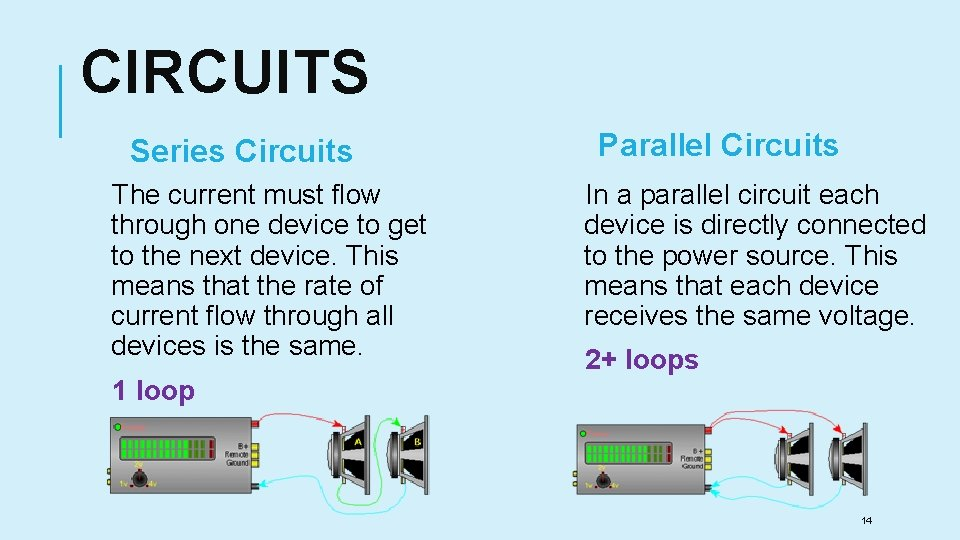CIRCUITS Series Circuits The current must flow through one device to get to the next device. This means that the rate of current flow through all devices is the same. 1 loop Parallel Circuits In a parallel circuit each device is directly connected to the power source. This means that each device receives the same voltage. 2+ loops 14CIRCUITS Series 1. Has a single loop for electrons to travel round 2. Components are connected one after another Parallel 1. Has two or more paths for electrons to flow down 2. Current is shared between the branches 3. Current has to travel through all components 15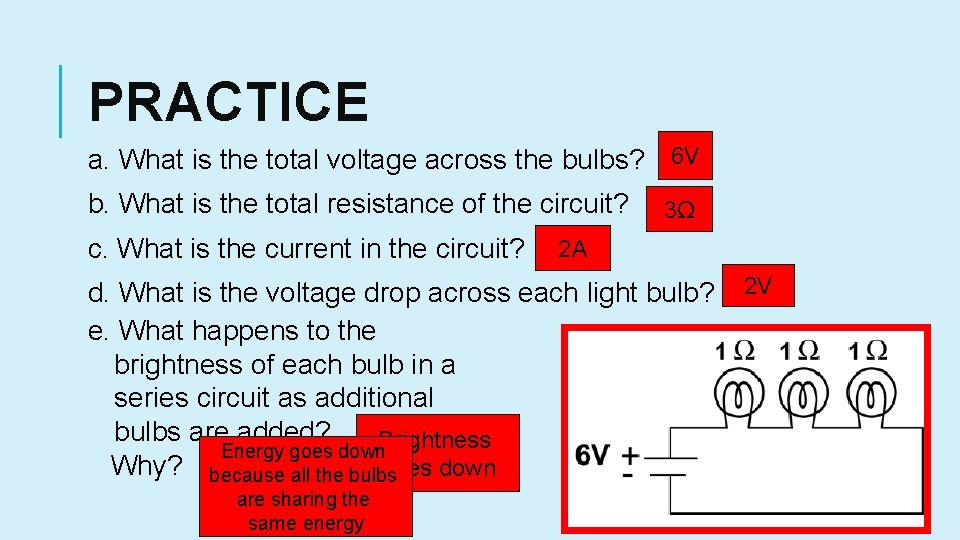PRACTICE a. What is the total voltage across the bulbs? 6 V b. What is the total resistance of the circuit? c. What is the current in the circuit? 3Ω 2 A d. What is the voltage drop across each light bulb? e. What happens to the brightness of each bulb in a series circuit as additional bulbs are added? Brightness Energy goes down Why? because all the bulbs goes down are sharing the same energy 2 V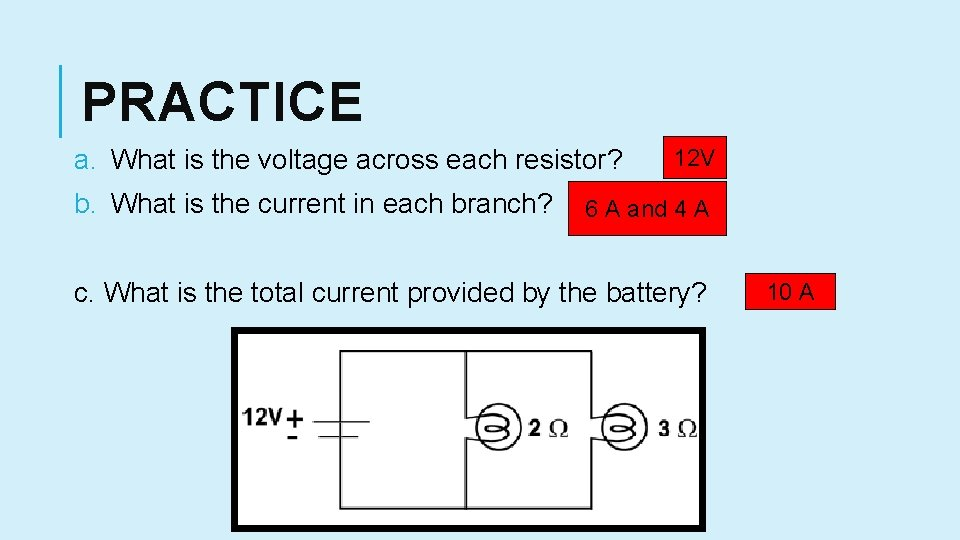PRACTICE a. What is the voltage across each resistor? b. What is the current in each branch? 12 V 6 A and 4 A c. What is the total current provided by the battery? 10 A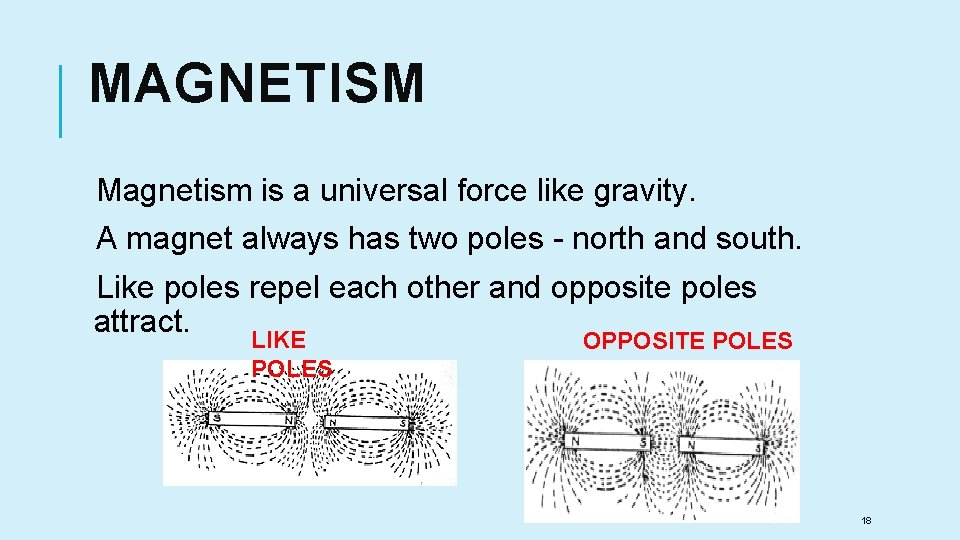MAGNETISM Magnetism is a universal force like gravity. A magnet always has two poles - north and south. Like poles repel each other and opposite poles attract. LIKE OPPOSITE POLES 18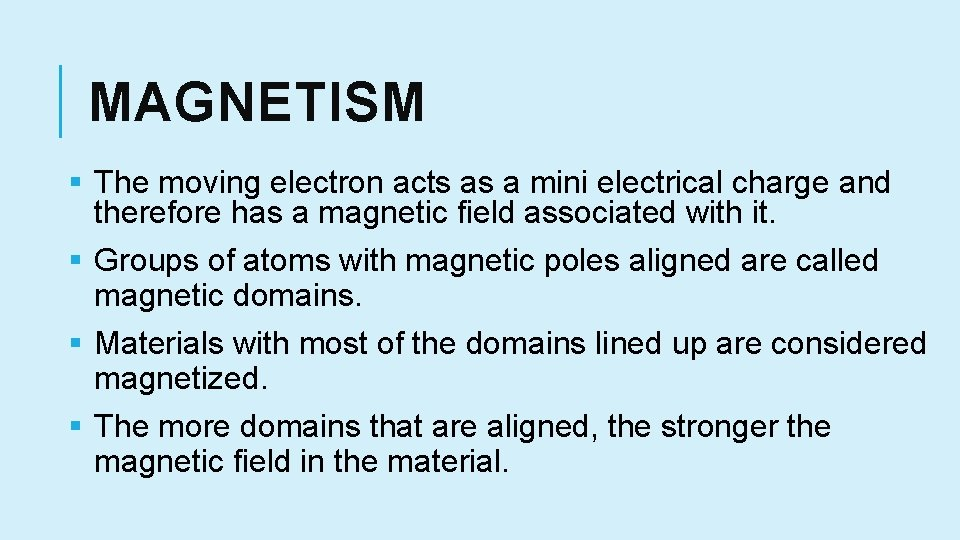MAGNETISM § The moving electron acts as a mini electrical charge and therefore has a magnetic field associated with it. § Groups of atoms with magnetic poles aligned are called magnetic domains. § Materials with most of the domains lined up are considered magnetized. § The more domains that are aligned, the stronger the magnetic field in the material.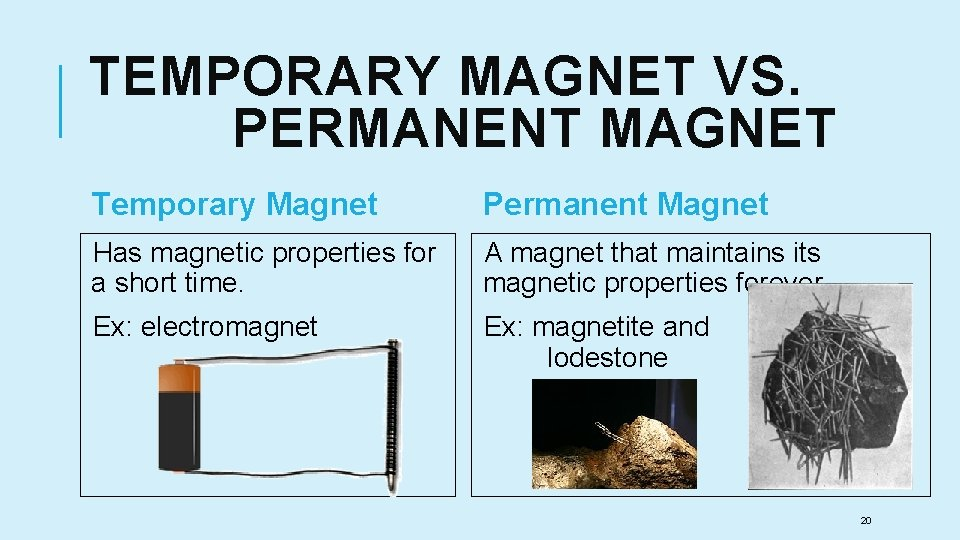TEMPORARY MAGNET VS. PERMANENT MAGNET Temporary Magnet Permanent Magnet Has magnetic properties for a short time. A magnet that maintains its magnetic properties forever. Ex: electromagnet Ex: magnetite and lodestone 20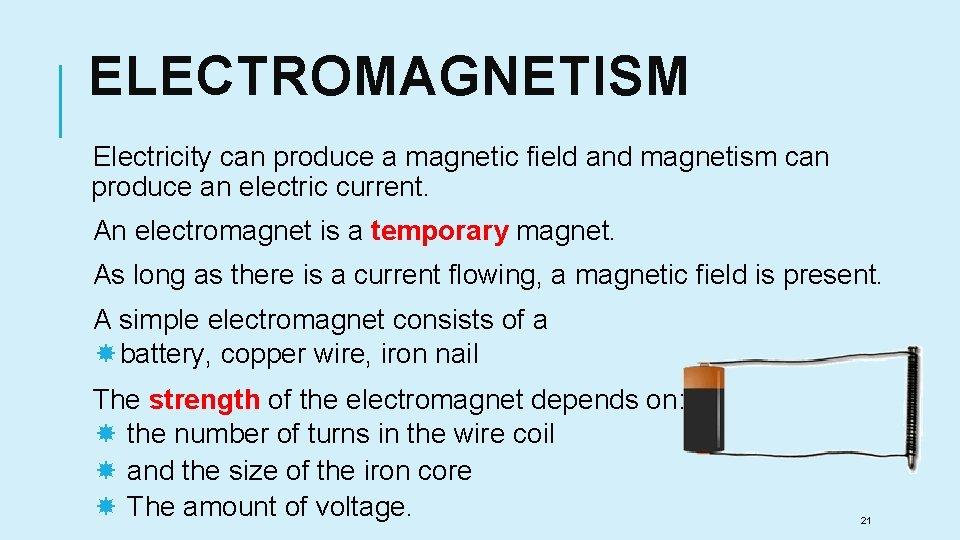ELECTROMAGNETISM Electricity can produce a magnetic field and magnetism can produce an electric current. An electromagnet is a temporary magnet. As long as there is a current flowing, a magnetic field is present. A simple electromagnet consists of a battery, copper wire, iron nail The strength of the electromagnet depends on: the number of turns in the wire coil and the size of the iron core The amount of voltage. 21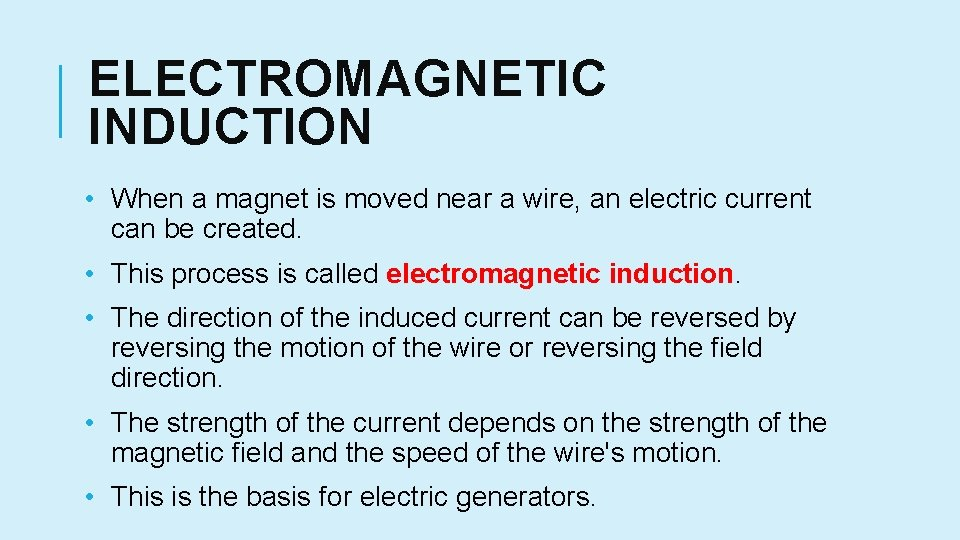ELECTROMAGNETIC INDUCTION • When a magnet is moved near a wire, an electric current can be created. • This process is called electromagnetic induction. • The direction of the induced current can be reversed by reversing the motion of the wire or reversing the field direction. • The strength of the current depends on the strength of the magnetic field and the speed of the wire's motion. • This is the basis for electric generators.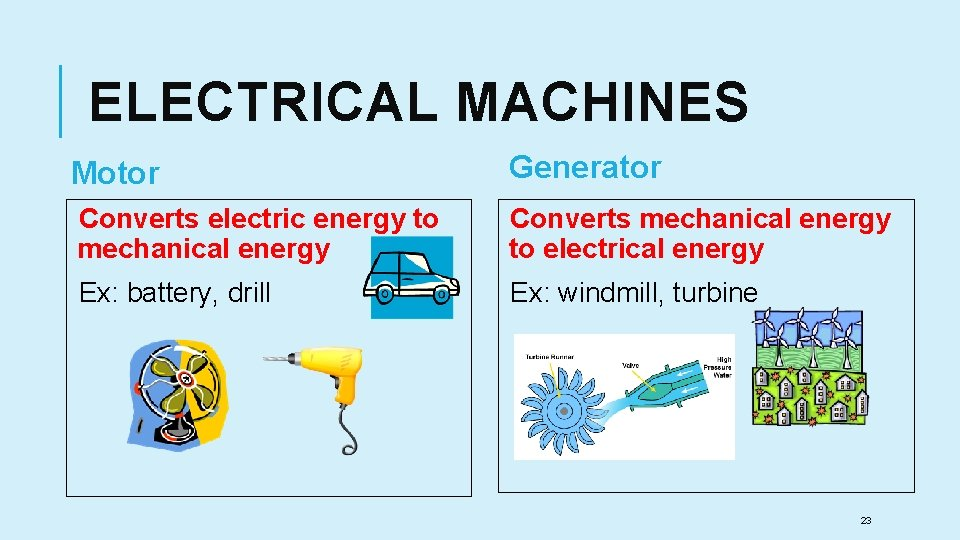ELECTRICAL MACHINES Motor Generator Converts electric energy to mechanical energy Converts mechanical energy to electrical energy Ex: battery, drill Ex: windmill, turbine 23